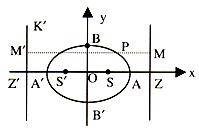# Why ellipse has two foci?

• I
• parshyaa

#### parshyaa

i think there is only one focus of ellipse and we can draw a ellipse with a single focus. please correct my thinking.

How would such an ellipse look like? Did you draw one, and if yes, how?

There are ellipses where both focal points are at the same place, but this special case has a different name which is usually preferred.

•fresh_42
i think there is only one focus of ellipse and we can draw a ellipse with a single focus. please correct my thinking.
Your thinking makes no sense. You may be thinking of a parabola. If you are really thinking of an ellipse, then you just need to study them more.

•parshyaa
so please explain me why ellipse has two focus

so please explain me why ellipse has two focus
If you spend any time studying the math of the ellipse it is very obvious why it has to focii. It's all there in the math and it's not hard. I'm puzzled by your confusion. Have you used the equation of an ellipse to draw one?

there are two definitions of ellipse one is the sum of the distance of a point from two foci is constant and another one is related to eccentricity. i was confused with the proof of the equation of ellipse using second definition because they have used only one foci and one directrix, which made me confused that why there are two foci, but now i got it. thank you my friends. this is the reason why i love physics forum

there are two definitions of ellipse one is the sum of the distance of a point from two foci is constant and another one is related to eccentricity. i was confused with the proof of the equation of ellipse using second definition because they have used only one foci and one directrix, which made me confused that why there are two foci, but now i got it. thank you my friends. this is the reason why i love physics forum
Ah, I see your confusion now. Just keep in mind that it is sometimes true that you can use different forms to describe the same thing but if they are indeed describing the same thing, then they have to be equivalent.

•parshyaa
There is no focal point involved in the definition via ##\frac{x^2}{a^2} + \frac{y^2}{b^2}=1##. You can calculate the two focal points based on that equation, but that needs some work.

•parshyaaThen we have SA = e AZ ... (1)

And SA' = e A'Z ... (2)

.·. A and A' lie on the ellipse.

Let AA' = 2a and take O the midpoint of AA' as origin. Let P(x, y) be any point on the ellipse referred to OA and OB as co-ordinate axis.
Then from figure it is evident that

AS = AO - OS = a - OS

AZ = OZ - OA = OZ - a

A'S = A'O + OS = a + OS

A'Z = OZ + OA' = OZ + a

Substituting these values in (1) and (2), we have

a - OS = e (OZ - a) ... (3)

a + OS = e (OZ + a) ... (4)

Adding (3) and (4), we get

2a = 2 e OZ

Or OZ = a/e ... (5)

Subtracting (3) from (4), we get

2 OS = 2ae => OS = ae ... (6)

.·. The directrix MZ is x = OZ = a/e and the co-ordinate of the focus S are (OS, 0) i.e. (ae, 0). Now as P(x, y) lies on the ellipse.

So we get

SP = e PM or SP2 = e2 PM2

(x - ae)2 + y2 = e2 [OZ - x co-ordinate of P]2

=> (x - ae)2 + y2 = e2 [a/e - x]2 = (a - ex)2 ... (7)

=> x2 + a2e2 - 2axe + y2 = a2 + e2x2 - 2aex

or x2/a2 + y2/a2(1-e2) = 1 [Dividing each term by a2 (1 - e2)]

or x2/a2 + y2/b2 = 1 where b2 = a2 (1 - e2)
in this proof they have used only one focus, i know that from both the focus equation will be same. but this made me confused to treat ellipse having only one true focus.

from this proof how can we say that ellipse has two foci

For the ellipse, it does not matter what you use in a proof. Also, you indirectly used the symmetry of the ellipse, and the other focus is symmetric to the first one.

•parshyaa
yes right

i think there is only one focus of ellipse and we can draw a ellipse with a single focus. please correct my thinking.
The requirement of two foci comes from the definition. The set of points in a plane whose sum of distance from two fixed points are equal. Said better in the first paragraph of this article: https://en.wikipedia.org/wiki/Derivation_of_the_Cartesian_form_for_an_ellipse [Broken]

Last edited by a moderator:
•parshyaa
How would such an ellipse look like? Did you draw one, and if yes, how?

There are ellipses where both focal points are at the same place, but this special case has a different name which is usually preferred.
Now that this topic has be discussed rather well, we can state that the name mfb refers to is "circle".

•symbolipoint, parshyaa and mfb